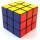# Cube containers

Two containers shaped of cube with edges of 0.7 m and 0.9 m replace a single cube so that it has the same volume as the original two together. What is the length of the edges of the new cube?

Result

a =  1.023 m

#### Solution:Leave us a comment of example and its solution (i.e. if it is still somewhat unclear...):

Showing 0 comments:Be the first to comment!#### To solve this example are needed these knowledge from mathematics:

Tip: Our volume units converter will help you with converion of volume units.

## Next similar examples:

1. Two boxes-cubesTwo boxes cube with edges a=38 cm and b = 81 cm is to be replaced by one cube-shaped box (same overall volume). How long will be its edge?
2. Water levelThe glass container has a cuboid shape with dimensions of the bottom 24 cm and 12 cm. The height of water is 26 cm. Calculate the volume of the body, which are sunk into the water if the water level rise by 3 cm.
3. Cube 9What was the original edge length of the cube if after cutting 39 small cubes with an edge length 2 dm left 200 dm3?
4. Cube basicsHow long is the edge length of a cube with volume 23 m3?
5. Cube and waterHow many liters of water can fit into a cube with an edge length of 0.11 m?
6. Cube cornersThe wooden cube with edge 64 cm was cut in 3 corners of cube with edge 4 cm. How many cubes of edge 4 cm can be even cut?
7. CubesCube, which consists of 27 small cubes with edge 4 dm has volume:
8. Cube cornersFrom cube of edge 14 cm cut off all vertices so that each cutting plane intersects the edges 1 cm from the nearest vertice. How many edges will have this body?
9. Cube 7Calculate the volume of a cube, whose sum of the lengths of all edges is 276 cm.
10. Perfect cubesSuppose a number is chosen at random from the set (0,1,2,3,. .. ,202). What is the probability that the number is a perfect cube?
11. The volume 2The volume of a cube is 27 cubic meters. Find the height of the cube.
12. Cube volumeThe cube has a surface of 384 cm2. Calculate its volume.
13. For thinkingsThe glass cube dive into the aquarium, which has a length of 25 cm, width 20 cm and height of 30 cm. Aquarium water rises by 2 cm. a) What is the volume of a cube? b) How many centimeters measure its edge?
14. RainfallThe annual average rainfall in India was in Cherrapunji in the year 1981 26 461 mm. How many hectoliters of water fell on 1 m2? Would fit this amount of water into a cube of three meters?
15. Volume of ballFind the volume of a volleyball that has a radius of 4 1/2 decimeters. Use 22/7 for π
16. Sphere growthHow many times grow volume of sphere if diameter rises 10×?
17. Sphere fallHow many percent fall volume of sphere if diameter fall 10×?